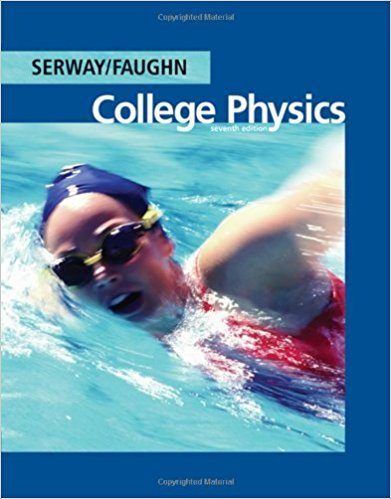×
Get Full Access to College Physics - 7 Edition - Chapter 18 - Problem 18.4
Get Full Access to College Physics - 7 Edition - Chapter 18 - Problem 18.4

×

# A 9.0-resistor and a 6.0-resistor are connected in seriesISBN: 9780495113690 154

## Solution for problem 18.4 Chapter 18

College Physics | 7th Edition

• Textbook Solutions
• 2901 Step-by-step solutions solved by professors and subject experts
• Get 24/7 help from StudySoup virtual teaching assistantsCollege Physics | 7th Edition

4 5 1 265 Reviews
21
4
Problem 18.4

A 9.0-resistor and a 6.0-resistor are connected in series with a power supply. (a) The voltage drop across the 6.0-resistor is measured to be 12 V. Find the voltage output of the power supply. (b) The two resistors are connected in parallel across a power supply, and the current through the 9.0-resistor is found to be 0.25 A. Find the voltage setting of the power supply.

Step-by-Step Solution:
Step 1 of 3

• System o Objectsinit o Forcesonitfromoutside(external) Fd Forceand Ability“todo Positivework Displacementare stuff”increases done parallel Fd Forceand Ability“todo Negativework Displacementare stuff”decreases done anti-parallel F Forceand Ability“todo Zeroworkdone Displacementare stuff”is d perpendicular uncharged W=±Fd Joules=Newtonsmeters (J)=(N)(m) Work=Forcedisplacement • ASystemHas: o Theobjectbeingdisplaced. o

Step 2 of 3

Step 3 of 3

##### ISBN: 9780495113690

College Physics was written by and is associated to the ISBN: 9780495113690. The answer to “A 9.0-resistor and a 6.0-resistor are connected in series with a power supply. (a) The voltage drop across the 6.0-resistor is measured to be 12 V. Find the voltage output of the power supply. (b) The two resistors are connected in parallel across a power supply, and the current through the 9.0-resistor is found to be 0.25 A. Find the voltage setting of the power supply.” is broken down into a number of easy to follow steps, and 66 words. This full solution covers the following key subjects: power, supply, resistor, voltage, Find. This expansive textbook survival guide covers 30 chapters, and 2135 solutions. The full step-by-step solution to problem: 18.4 from chapter: 18 was answered by , our top Physics solution expert on 11/15/17, 04:28PM. This textbook survival guide was created for the textbook: College Physics, edition: 7. Since the solution to 18.4 from 18 chapter was answered, more than 270 students have viewed the full step-by-step answer.

Unlock Textbook Solution